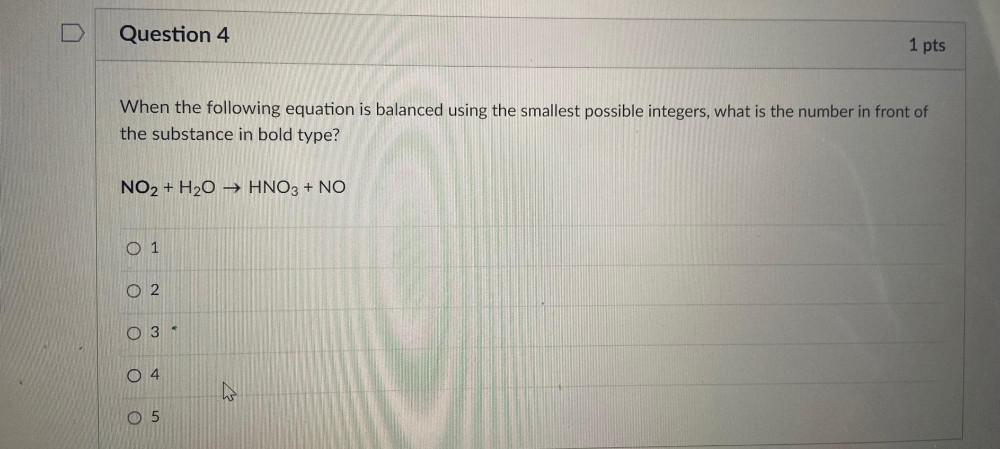Question:

# Question 4 1 pts When the following equation is balanced using the smallest possible integers, what is the number in front of thQuestion 4 1 pts When the following equation is balanced using the smallest possible integers, what is the number in front of the substance in bold type? NO2 + H2O → HNO3 + NO 0 1 O 2 A O 3 O4 th O 5